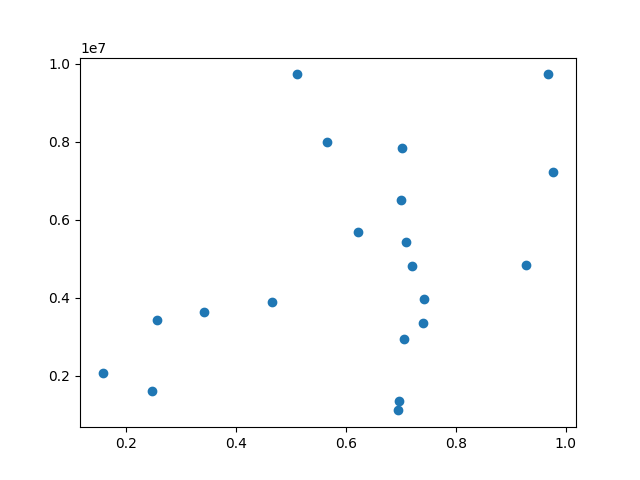# Coords Report¶

Override the default reporting of coords.import matplotlib.pyplot as plt
import numpy as np

def millions(x):
return '\$%1.1fM' % (x*1e-6)

# Fixing random state for reproducibility
np.random.seed(19680801)

x = np.random.rand(20)
y = 1e7*np.random.rand(20)

fig, ax = plt.subplots()
ax.fmt_ydata = millions
plt.plot(x, y, 'o')

plt.show()


Keywords: matplotlib code example, codex, python plot, pyplot Gallery generated by Sphinx-Gallery